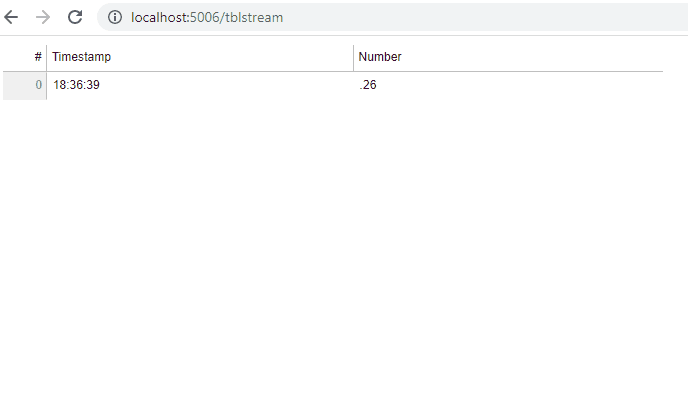# Streaming to the top of the datatable

Hello!

I am trying to stream data to a DataTable and it seems I am missing some basic piece of knowledge. Every second I stream a new datapoint to the table. The point contains current timestamp and some random number. My problem is that the new datapoint gets appended to the end of the table. Unless the users clicks the header and sorts the table all the new points will be appended to the end. Is it possible somehow to stream data to the top of the table? Or to programmatically enforce the sorting of the table?

The end result should be that without user interaction the table should just show newest datapoint at the top of the table.

I know I could sort the whole dataset myself and then do source.data = data. But this will be far less efficient than simple stream of one point.

Here is an example code that currently streams to the bottom of the table. Is there something I can do to force the new point to be at top of the table?

``````import bokeh.models
import bokeh.plotting

import pandas as pd
import numpy as np

doc = bokeh.plotting.curdoc()

source = bokeh.models.ColumnDataSource(data=dict(
timestamp=[pd.Timestamp.now()],
number=[np.random.normal()],
))

columns = [
bokeh.models.TableColumn(
field="timestamp",
title="Timestamp",
formatter=bokeh.models.DateFormatter(format="%H:%M:%S")),
bokeh.models.TableColumn(
field="number",
title="Number",
formatter=bokeh.models.NumberFormatter(format=".00")),
]

table = bokeh.models.DataTable(
source=source,
columns=columns
)

def stream_point():
source.stream(dict(timestamp=[pd.Timestamp.now()], number=[np.random.normal()]))

``````

I see what you’re getting at with the “inefficiency.” You’re right, you don’t need to sort the data each time you add a data point → this is only by virtue of knowing that the newest datapoint should always go “on top”. But the source.patch on index 0 method won’t work here because you don’t want to replace values at certain indices, you want to add a new 0th index and shift all the other indices “down”. That index shift is the only remaining inefficiency and I don’t think there’s any way to avoid it… but that’s wayyyyy less expensive than sorting each time. So let’s do that?

A pandas-based solution would be as follows. I really find the .to_df() method from the CDS class super handy.

``````def stream_point():
#get existing data as a df
df = source.to_df()
#make your new row of data and append to the top of the df
row = pd.DataFrame(data=dict(timestamp=[pd.Timestamp.now()], number=[np.random.normal()]))
df = pd.concat([row,df])
#reassign the datasource using the df
source.data = {c:df[c].tolist() for c in df.columns}
``````Hope this is sufficient and we get to see what you’re building sometime soon!

2 Likes

Thank you once more @gmerritt123 !

This is a very helpful answer and solves my problem. I am mostly grateful for understanding that I am not missing some special property of the DataTable.

Actually, I also got another idea and it seems to be working. In the following code I add an invisible button. Attach a CustomJS callback to it and force this callback by changing the label of the button. The JS callback simulates user click on the header so that we get the table sorted on startup without need for user interaction.

``````import bokeh.models
import bokeh.plotting
import bokeh.layouts
from bokeh.models.callbacks import CustomJS

import pandas as pd
import numpy as np

doc = bokeh.plotting.curdoc()

source = bokeh.models.ColumnDataSource(data=dict(
timestamp=[pd.Timestamp.now()],
number=[np.random.normal()],
))

columns = [
bokeh.models.TableColumn(
field="timestamp",
title="Timestamp",
formatter=bokeh.models.DateFormatter(format="%H:%M:%S"),
default_sort="descending"),
bokeh.models.TableColumn(
field="number",
title="Number",
formatter=bokeh.models.NumberFormatter(format=".00")),
]

table = bokeh.models.DataTable(
source=source,
columns=columns
)

def stream_point():
source.stream(dict(timestamp=[pd.Timestamp.now()], number=[np.random.normal()]))

js_callback = CustomJS(code="""

for (const span of document.querySelectorAll("span")) {
if (span.textContent.includes("Timestamp")) {
span.parentElement.click();
}
}

""")

button = bokeh.models.Button(label="Not sorted");
button.visible = False
button.js_on_change("label", js_callback);

def sort_table():
button.label = "Sorted"